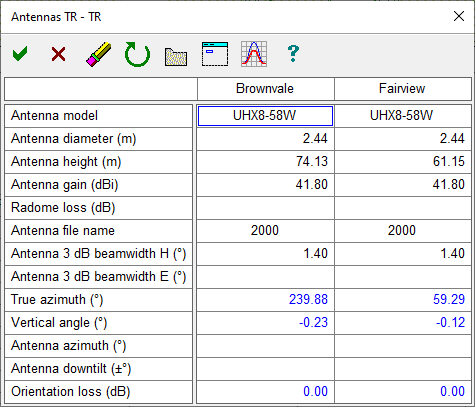## Antenna Data Entry Form

Click on either the Site 1 or 2 antenna icon to access the antenna data entry form. The format for land mobile applications includes the antenna gain in both dbi and dbd units but does not include the radome loss or antenna diameter. The caption on the data entry form show the antenna configuration. Data can be entered from an antenna lookup table or the from the antenna data index.

.Antenna Model Information only Antenna Diameter This is required for near field calculations in rectangular passive repeater applications. Antenna Height Antenna heights can be entered directly in this data entry form but are normally calculated in the Antenna Heights module. If you change the value here, be sure to reassess the clearance or diffraction loss. Antenna Gain (dBi) Antenna gains are a required entry Radome Loss Optional entry. On high frequency systems, where rain attenuation is a factor, the value should reflect the wet radome loss. Code The antenna code is required for interference and orientation loss calculations. Note that the code cannot be directly entered and must be loaded from a lookup table or antenna data index. Antenna 3 dB Beamwidth Information only. This will become the default value for antenna discrimination calculations in the Multipath - Reflections module. True Azimuth This is calculated from the geographic coordinates and cannot be edited. Vertical Angle This is calculated using an earth radius factor of K = 4/3. A path profile must be available. For antenna configurations with more than one antenna, the vertical angle is calculated for the first antenna only and is assumed to be the same for the others. Antenna Azimuth This will be primarily used for the hub site on point to multi point applications where a fixed antenna azimuth interconnects with remote antennas. The antenna discrimination is determined from the difference of the true azimuth and the antenna azimuth. If this entry is blank (F3 key), the horizontal antenna orientation is assumed to correspond to the true azimuth. Antenna Downtilt This is the corresponding fixed vertical angle at a hub site in a point to multipoint application. Downtilt is positive below the horizontal. The antenna discrimination is determined from the difference of the actual vertical angle and the antenna downtilt. In a microwave application, the vertical angle is calculated at K = 4/3 and line of sight is assumed. In a land mobile application, the vertical angle will be the horizon angle on an obstructed path. If this entry is blank (F3 key), the vertical antenna orientation is assumed to correspond to the vertical angle. Note that in a land mobile application the antenna boresight is always horizontal. Orientation Loss This the antenna response to the off azimuth and off elevation and off vertical angles above. Refer to the technical reference at the end of this section for details. An antenna code must be available for this calculation.